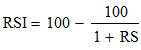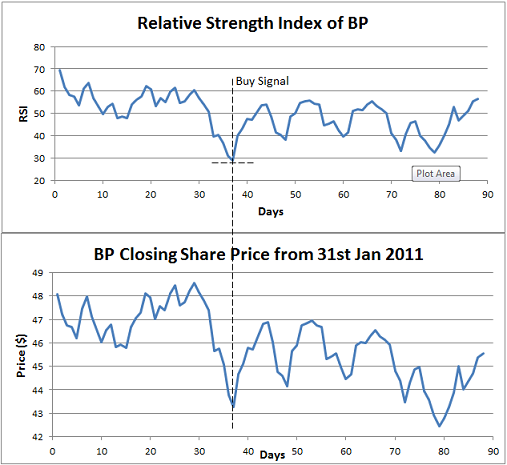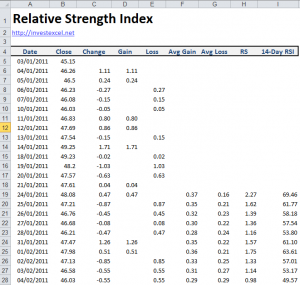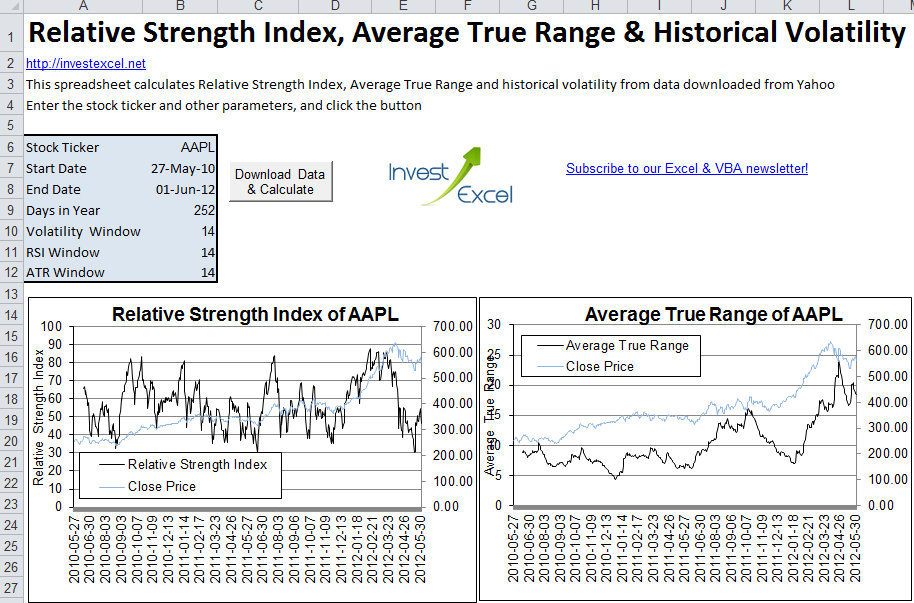# Relative Strength Index: Become a Better Trader

The Relative Strength Index helps you decide if a stock is overbought or oversold. Learn about it, and get an Excel spreadsheet that calculates RSI of a stock based on its ticker symbol.

The momentum of the stock market is rather like a ball that’s thrown upwards. Once the ball is thrown, it keeps on going until it reaches its apex, and then it starts falling. The principles that physicists employ to measure the velocity and acceleration of the ball are similar to those use to gauge price action.

Stock market momentum is measured by several techniques, and the Relative Strength Index (or RSI) is one of the most popular. It’s a momentum-based oscillator and is a widely used technical analysis tool. It was introduced by J. Wells Wilder in New Concepts In Trading Systems. RSI compares recent upwards movements  to recent downwards movements in the closing price of a stock.

## How to Calculate Relative Strength Index

RSI is defined by this equation.where RS is the Relative Strength Factor. RS is a moving average – this is either an exponential moving average, or an equally-weighted mean. The averaging window is usually  14 days, but is sometimes more or less. Assuming an equally-weighted mean, the initial value of RS is calculated as follows.

• First average gain: looking back over the previous two weeks, you note those days in which the stock has finished up. You calculate the average gain over all of these days.
• First average loss: looking back over the previous two weeks, you note those days in which the stock has finished down. You calculate the average loss over all of these days.

The first value of RS is the first average gain divided by the first average loss.

• All other values of average gain: [(previous average gain × 13 + current gain) / 14]
• All other values of average loss: [(previous average loss × 13 + current loss) / 14]

All other values of RS are simply the first value divided by the second value.

The moving average smooths the impact of large price movements

## How Do You Interpret Relative Strength Index?

RSI varies between 0 and 100. A stock is generally considered overbought if RSI moves above 70, or oversold if its RSI moves below 30.

If the stock price reaches new highs, but the RSI does not rise above its  previous high, then the stock price is due to fall.

When the RSI move above 50, the average gains outweigh the average losses; this is regarded as bullish. When the RSI falls below 50, the average losses outweigh the average gains; this is regarded as bearish.

Lets look at a specific example of how RSI can be used to generate buy and sell signals. This chart gives the share price and RSI of BP for the 90 days from 3rd January 2011.At around 38 days, the RSI touches 30. This generates a buy signal and the share price rebounds upwards as buyers enter the market.

## Calculate Relative Strength Index in Excel

This Excel spreadsheet demonstrates how you can calculate RSIThe spreadsheet uses data for BP from 3rd January 2011 to 27th May 2011 (which was retrieved with this spreadsheet), and implements the calculation steps given above. All the calculations are manual.

This spreadsheet, however, is a lot more clever. It automatically calculates and plots RSI based on data downloaded from Yahoo Finance. It also plots the Average True Range , MACD, 12- and 26 day EMA, and the historical volatility.Simply type in a stock ticker, two dates, and the number of days in averaging period. After you click a button, the spreadsheet downloads stock quotes from Yahoo finance, and then calculates and plots RSI and ATR. As a bonus, it also plots the historical volatility. Everything is automated in VBA.### 27 thoughts on “Relative Strength Index: Become a Better Trader”

1. I am getting a ‘Compile error in hidden module Mdlwin32API’ error.Any idea what that might be ?

• I noticed this error a couple of days ago on 64-bit Excel (I used 32-bit Excel to develop the spreadsheet). I’ve modified the code and re-uploaded. Could you re-download the file and try again please?

2. I’m still seeing the error with excel 2007 32bit

• hmmm….I’ve tested the spreadsheet on Excel 2010 32-bit and 64-bit, and it works fine. I’ll see if I can find Excel 2007 to test the spreadsheet one

3. I get the same error on 2007. Any logs or anything I can send you to help troubleshoot?

• Try version, let me know if it works. The original spreadsheet works fine on Excel 2010 64 and 32 bit for me…

4. Hi Samir, I’m getting the very same error with Excel 2007…

• See my reply to Gary

5. I get the following msg as I try to install the RSI spreadsheet on Excel 2007 (32 or 64):
‘There was a problem sending the command to the program”
I am not a computer savy person, so please forgive me for asking this otherwise simple question.
Thanks.
monypro

• Hello Vasanth

This is the latest version of the spreadsheet. I’ve tested it on Excel 2010 32 and 64 bit (it works as expected).

Unfortunately I don’t have Excel 2007 to test the spreadsheet against.

• Thanks, Samir.
Vasanth

6. Thank you for the excellent program.

How can I edit the VBA to ensure that the excel sheet pulls through information for South African stocks? i.e. Mr Price (MRP – JSE)

• I’d have to recode the VBA to pull data from Google Finance (who offer data for South African stocks, and recognizes PINK:MRPZY). The spreadsheet currently uses Yahoo Finance.

7. Hi,

Thanks for sharing.

I compared the RSI values in yahoo finance and in your excel file and they seem to be different.
Am I missing something?
The way I compared (aapl) is: in yahoo finance taking the mouse pointer to, for example, february 19 2013.
The value on the RSI indicator is 51.15. However in the spreadsheet is 37.70
I checked that the close price is the same. It matches. So it seems that it’s taking the data correctly.
Any ideas?

• I looked into this. The difference creeps in when calculating the average gain and average loss. My spreadsheet uses an exponential moving average. Yahoo uses an equally-weighted arithmetic mean.

Both methods are valid (see http://en.wikipedia.org/wiki/Relative_strength_index)

When I alter the spreadsheet to use an arithmetic mean, the spreadsheet and Yahoo give similar results

8. Hi Samir,

thanks for responding and sorry for the huge delay!
I’m now comparing yahoo finance to the RSi at Reuters and http://stockcharts.com
Yahoo finance seems to be different than all the rest of the charts.
However yahoo finance RSI seems to be more accurate in terms of signals to buy/sell.

Do you know what could possibly be the reason?
Do you think Reuters and Stockcharts use the exponential RSI same as you said before for your excel sheet?

9. Hi All,

Any Web API (something like ImportData(“http://finance.yahoo.com/d/quotes.csv…..), that can query’s today’s or any given date’s RSI ? It can be useful in desktop/web-based spreadsheet, for investors who bother to know today’s RSI of a stock.

Thanks.

SH Chong

10. Hi Samir,

I’m using IB TWS ActiveX excel to trade with IB. I would like to import real time RSI value from TWS into the ActiveX excel. How can I possibly do that?

Best regards,
Risalde

11. Brilliant!!

I tried a few tickers, and all seem to work fine. Thanks a great bunch.

Barring the RSI graph, I am not sure how to use the other graphs in the investing decisions, though.

Ram

12. Hi Samir:
On the MACD chart, anyway you can move the dates and histogram below the chart so we can see the signals more clearly? Thanks, Fred

13. Samir:
Looking at JPM, the RSI as of 2/24 is just below 50 (so bearish), and the MACD has just crossed the signal line, indicating a sell signal, right? My question is: Is the MACD more accurate in terms of giving a precise sell (or buy) indication than RSI? You indicate above a stock is overbought above an RSI of 70, so I think some of us are confused if it didn’t hit 70 but has declined below 50 if that is our signal to start paying attention to the MACD for a signal, as it might be coming? Thanks, Fred

14. Samir:
I am having trouble pulling out commodities from Yahoo. Any suggestions?

• I don’t know if Yahoo has comprehensive data for commodities. You might try locating another data provider (with programmatic access to prices). Quandl maybe if you want free data

15. When I try to view code I am asked for a password… Would you be able to provide the password to view and edit the VBA?

Many thanks

16. Hi Samir,

Great job. It’s better if it can generate the RSI for multiple stocks at the same time.
Unfortunately it is password protected and does not allow to modify or add additional VBA; otherwise I’ll do it myself to meet my needs.

Regards,

17. hi Samir,
let me first say that I find your spreadsheets/VBA code really useful and well done.
there is only one thing I am not 100% so I would like to check with you before going ahead: in your RSI manual calculation file you use one an “average” formula in cell F19 and G19, but then you change it in the cells below. Not sure why but if I extend those 2 formulae to all cells below then I end up with very different RSIs. So I’m wondering which formula I should use.
thanks a lot,
Andrea